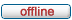# forum.alglib.net

ALGLIB forum
 It is currently Sat Jul 02, 2022 4:56 am

 All times are UTC

### Forum rules

1. This forum can be used for discussion of both ALGLIB-related and general numerical analysis questions
2. This forum is English-only - postings in other languages will be removed.

 Print view Previous topic | Next topic
Author Message
 Post subject: spline1dbuildlinearPosted: Mon Jan 30, 2017 5:21 amJoined: Tue Jan 17, 2017 11:16 pm
Posts: 10
Dear Sergey,

I also have another query in regards to the spline1dbuildlinear(AX, AY,spline) function.

As I understand that the function currently only accepts array of physical values, if I have X nodes = [0, 1/12, 3/12, 1/2, 1, 2, 5, 10,15, 20] and Y[i] = X[i]*log(p(X[i],a,b,var,r0)), how do I store the values of Y[i] as array to apply to the spline1dbuildlinear(AX, AY,spline) function ?

Your help is much appreciated. Many thanks.

Cheers,
Van

TopPost subject: Re: spline1dbuildlinearPosted: Mon Jan 30, 2017 10:11 amSite Admin

Joined: Fri May 07, 2010 7:06 am
Posts: 873
You should calculate Y[] using formula given by you and pass these values to spline constructor. It is the only way to build spline - to specify exact numerical values. It can't be built using some symbolic expression like yours.

TopPost subject: Re: spline1dbuildlinearPosted: Mon Jan 30, 2017 11:23 amJoined: Tue Jan 17, 2017 11:16 pm
Posts: 10
Dear Sergey,

I try to build it like this and it works. However, it looks clumsy.

Y = pow(X,1)*log(p(X,a,b,var,r0));
Y = pow(X,1)*log(p(X,a,b,var,r0));
Y = pow(X,1)*log(p(X,a,b,var,r0));
Y = pow(X,1)*log(p(X,a,b,var,r0));
Y = pow(X,1)*log(p(X,a,b,var,r0));
Y = pow(X,1)*log(p(X,a,b,var,r0));
Y = pow(X,1)*log(p(X,a,b,var,r0));
Y = pow(X,1)*log(p(X,a,b,var,r0));
Y = pow(X,1)*log(p(X,a,b,var,r0));
Y = pow(X,1)*log(p(X,a,b,var,r0));

Is there a better was=y to handle this?

Cheers,
Van

TopPost subject: Re: spline1dbuildlinearPosted: Mon Jan 30, 2017 3:05 pmSite Admin

Joined: Fri May 07, 2010 7:06 am
Posts: 873
...are you talking about for() loop? :)

TopPost subject: Re: spline1dbuildlinearPosted: Tue Jan 31, 2017 4:36 amJoined: Tue Jan 17, 2017 11:16 pm
Posts: 10
Dear Sergey,

Thanks for the response.

I did this in a for() loop. However, how do I code the for() loop in the spline1dbuildlinear (Array X, Array Y) for Y?

X[i] = [0 1/12, 1/4, ....20];
for (i =0; i < 10; i++){
Y[i] = pow(X[i],0)*log(B(X[i],a,b,c));
}

Many thanks.
Cheers,
Van

TopPost subject: Re: spline1dbuildlinearPosted: Tue Jan 31, 2017 11:19 amJoined: Tue Jan 17, 2017 11:16 pm
Posts: 10
Hi Sergey,

Its all good until next time. I put the function in the for() loop.

for (int n = 0; n<3; n++){

for (int i=0;i<10;i++){
Y[i] = pow(X[i],n)*log(p(X[i],a,b,var,r0));
//cout << setprecision(11)<< Y[i] << ",";
}

AY.setcontent(Y.size(), &(Y));

alglib::spline1dbuildcubic(AX, AY,spline); //alglib building the linear spline
cout << spline1dintegrate(spline,X) << ", ";

}
Thanks again.
Cheers,
Van

TopDisplay posts from previous: All posts1 day7 days2 weeks1 month3 months6 months1 year Sort by AuthorPost timeSubject AscendingDescending

 All times are UTC

#### Who is online

Users browsing this forum: No registered users and 4 guests

 You cannot post new topics in this forumYou cannot reply to topics in this forumYou cannot edit your posts in this forumYou cannot delete your posts in this forumYou cannot post attachments in this forum

Search for: